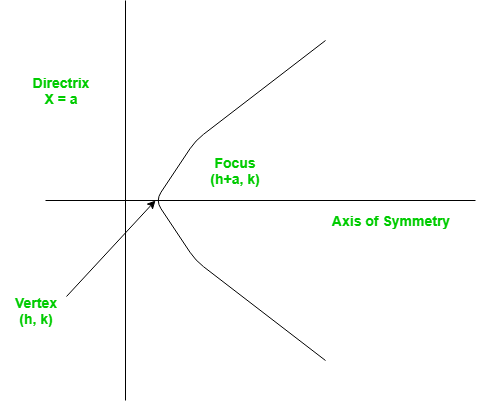# Python Program for Finding the vertex, focus and directrix of a parabola

• Last Updated : 03 Dec, 2018

A set of points on a plain surface that forms a curve such that any point on that curve is equidistant from the focus is a parabola.
Vertex of a parabola is the coordinate from which it takes the sharpest turn whereas a is the straight line used to generate the curve.The standard form of a parabola equation is. Given the values of a, b and c; our task is to find the coordinates of vertex, focus and the equation of the directrix.

Example –

```Input : 5 3 2
Output : Vertex:(-0.3, 1.55)
Focus: (-0.3, 1.6)
Directrix: y=-198
Consult the formula below for explanation.
```

## Recommended: Please try your approach on {IDE} first, before moving on to the solution.

 `# Python program to calculate Vertex, Focus and Directrix`` ` `def` `parabola(a, b, c):``    ``print` `(``"Vertex: ("` `, (``-``b ``/` `(``2` `*` `a)) , ``", "``        ``,(((``4` `*` `a ``*` `c) ``-` `(b ``*` `b)) ``/` `(``4` `*` `a)) , ``")"` `)``         ` `    ``print` `(``"Focus: ("` `, (``-``b ``/` `(``2` `*` `a)) , ``", "``        ``, (((``4` `*` `a ``*` `c) ``-` `(b ``*` `b) ``+` `1``) ``/` `(``4` `*` `a)) , ``")"` `)``     ` `    ``print` `(``"Directrix: y="``            ``, (``int``)(c ``-` `((b ``*` `b) ``+` `1``) ``*` `4` `*` `a ))    ``     ` `     ` `# main()``a ``=` `5``b ``=` `3``c ``=` `2`` ` `parabola(a, b, c)`` ` `# Contributed by _omg`

Output :

```Vertex:(-0.3, 1.55)
Focus: (-0.3, 1.6)
Directrix: y=-198
```

Please refer complete article on Finding the vertex, focus and directrix of a parabola for more details!

My Personal Notes arrow_drop_up